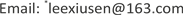AHP结合多元回归对影响大学生数学学习质量因素的实证分析<sup> </sup>1. 引言

2. 基于AHP对大学生数学质量的影响因素的分析2.1. 构建多层次结构模型

2.2. 一致性检验并确定各影响因素的全局权重

W = [ 0.078343 0.441436 0.166847 0.313374 ] ， λ max = 4.0079 C I = 0.0026 R I = 0.90 C R = 0.0029 W = [ 0.285714 0.285714 0.071429 0.214286 0.142857 ] ， λ max = 5.0 C I = 0 R I = 1.12 C R = 0

W = [ 0.277778 0.222222 0.222222 0.111111 0.166667 ] ， λ max = 5.0 C I = 0 R I = 1.12 C R = 0 W = [ 0.298468 0.203475 0.171101 0.326955 ] ， λ max = 4.228 C I = 0.0759 R I = 0.9 C R = 0.0843

， λ max = 3.0004 C I = 0.0002 R I = 0.58 C R = 0.0003

C层次总排序的一致性检验：

C I = ∑ 2 4 B i C I = 0.441436 × 0 + 166847 × 0 + 0.313374 × 0.0759 = 0.023785

R I = ∑ 2 4 B i R I = 0.441436 × 1.12 + 0.166847 × 1.12 + 0.313374 × 0.9 = 0.963314

C R = C I R I = 0.0247

D层次总排序的一致性检验：

C R = C I R I = 0.0003

Each factor affects the weigh

2.3. 各影响因素权重结果分析

3. AHP结合多元回归对影响大学生数学学习质量因素的实证分析

y = 61.551 + 0.197 x 1 + 0.215 x 2 − 0.136 x 3 − 2.067 x 4

x 1 ：复习课堂内容情况； x 2 ：回答问题跟上思路情况；

x 3 ：课前预习情况； x 4 ：考前学习时间。

Model summar

10.451d0.2040.19010.66151.724

y = 64.421 + 0.2 x 1 − 2.266 x 2 − 0.112 x 3 + 0.162 x 4

x 1 ：课堂内容当时理解程度； x 2 ：学习时间；

x 3 ：课前预习情况； x 4 ：回答问题跟上思路。

Model summar

40.418d0.1750.16110.85171.659
d.预测变量：(常量)，课堂内容当时理解程度，学习时间，课前预习情况，回答问题跟上思路；因变量：成绩

4. 研究建议